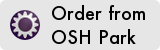# Lights Test Board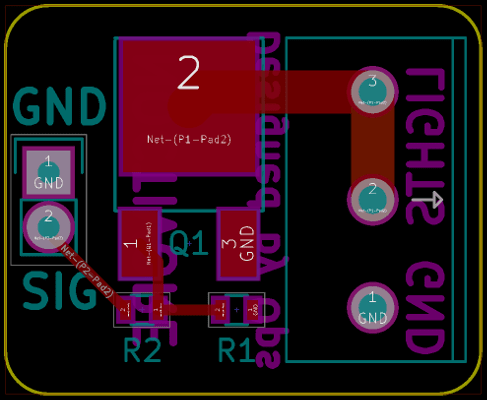Lights, motors, relays, and solenoids often operate on +12V DC and present a large electrical load.

Many microcontrollers today operate at +3.3V DC and can only source a relatively small current so a method is needed to switch a large load with the output of only a small microcontroller pin.

A MOSFET is often used to accomplish this type of task.

A MOSFET is a type of transistor as its acronym implies.
Don't let the fancy, complicated sounding acronym put you off though, they're really quite simple to use once you get past all of the elaborate sounding words.

The circuit above may look simple, after all it's only three parts (two of which are resistors) and a couple of connectors. How complex could it be?
If the length of this post is any indication, there is a lot more going on here than meets the eye.
To understand why this circuit works we'll need to demystify a few things...

## Perplexing Parlance

If you have ever tried do use a MOSFET before and were befuddled by all of the jargon, you are not alone.
While the terminology may have made sense in some bygone era, it now appears largely antiquated, contradictory, and confounding.
A few cases in point:

• The metal in the acronym MOSFET used to refer to the aluminum gate material but most gates are now polysilicon.
• The oxide meant to refer to the insulating layer can now be a variety of different dielectric materials.
• Charge does not necessarily flow from the `SOURCE` to the `DRAIN` (we'll cover these terms shortly) as the names might imply.
• N or P-Channel MOSFETS refer to the channel between the `SOURCE` and `DRAIN`, so an N-Channel MOSFET is made with a P-type substrate, and a P-Channel MOSFET with an N-type substrate.
• Naming conventions and capitalization are loosely enforced at best with terms such as N-Channel, n-channel, nMOSFET, nMOS, and NMOS all usually referring to the same thing.

So let's get our science hats on and unravel a bit of this mystery!

## Dizzying Descriptions

Much like its cousin the BJT the MOSFET can be used to amplify or switch electronic signals.

The main advantage of the MOSFET over other types of transistors is that it can often switch a large amount of current with only a very small amount of input (often less than 1mA), which is exactly what we'd like to do.
MOSFETs also happen to switch quite fast compared to some other transistors which is a nice bonus.

Let's look at a simplified diagram of a MOSFET:• The `GATE` is used to turn the MOSFET ON or OFF.
• The `SOURCE` and `DRAIN` serve as the input and output for whatever is being switched.
Be careful not assume that current will always flow from `SOURCE` to `DRAIN` as the names might imply.
The direction of current flow depends upon the type of MOSFET being used and can be quite confusing at first.
• The `BODY` of the MOSFET is typically internally connected to the `SOURCE` so you usually won't need to worry too much about it.

### Type, Channels, Modes, and Levels (To the tune of Head, Shoulders, Knees and Toes)

MOSFETs come in several different flavors.
The particular taste for our palate at the moment is the Power MOSFET.
Unlike virtually everything else about MOSFETs, this one does what it says and is designed for higher power applications such as switching lights or small DC motors.
Technically speaking, there are some physical differences in the construction of Power MOSFETs (the internal structure is vertical instead of planar) but this should not affect the way they are used in our application.

MOSFETs also come in a couple of different channels (N-channel and P-channel) as well as modes (depletion and enhancement).

Generally speaking, the following rules apply to MOSFETs:

• In an N-channel MOSFET conventional current flows from `DRAIN` to `SOURCE`.
• In a P-channel MOSFET conventional current flows from `SOURCE` to `DRAIN`.
• An enhancement mode MOSFET is OFF by default. (when the voltage between the `GATE` and the `SOURCE` is zero)
Enhancement mode MOSFETs are, by far, the most common.
• A depletion mode MOSFET is ON by default. (when the voltage between the `GATE` and the `SOURCE` is zero)
• An enhancement mode N-Channel MOSFET can be turned ON by pulling its `GATE` voltage higher than its `SOURCE` voltage.
• An enhancement mode P-Channel MOSFET can be turned ON by pulling its `GATE` voltage lower than its `SOURCE` voltage.
• In most circuits pulling an enhancement-mode MOSFET's `GATE` voltage towards its `DRAIN` voltage turns it ON.

One other critical parameter we need to concern ourselves with is the Threshold voltage or the `GATE`-`SOURCE` threshold voltage often expressed in datasheets as VGS(th).

• VGS (without the 'th') or the `GATE`-`SOURCE` voltage is simply the voltage between the `GATE` and `SOURCE` pins at any particular time (VG - VS).

In a nutshell, VGS(th) is the minimum voltage difference between the `GATE` and the `SOURCE` pins required to switch the MOSFET's gate ON or OFF depending upon the mode (enhancement or depletion respectively).
In many MOSFETs this value can be considerably higher than a typical microcontroller can provide.
While there certainly are tricks to making this work the simple solution is to chose a MOSFET whose threshold voltage is easily attained by a microcontroller.
This type of MOSFET is often called a Logic-level, or sometimes just Logic, MOSFET.

Below is an example section from a logic-level MOSFET datasheet: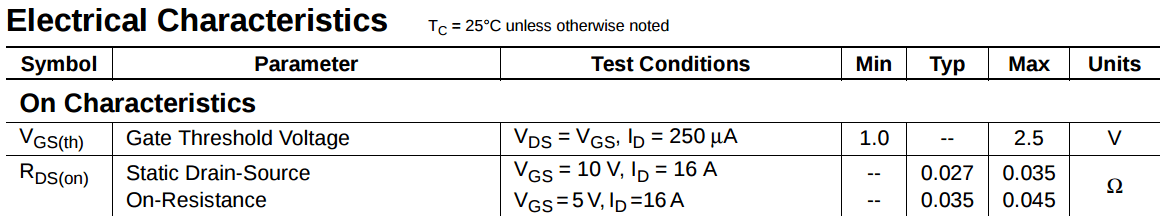This section shows the minimum (+1.0V) and maximum (+2.5V) VGS(th).
This is the minimum range at which the MOSFET is guaranteed to start to turn ON.
We can see from the Test Conditions that within this range the Drain Current (ID) is only 250 micro-amps which probably isn't enough to accomplish anything very useful.

When the `GATE`-`SOURCE` voltage is sufficiently above VGS(th) the MOSFET will be fully turned ON and current will be allowed to flow with very little resistance.
This is referred to as Saturation.
Saturation occurs when VGS > VGS(th) and the voltage between the `DRAIN` and the `SOURCE` (VDS) ≥ ( VGS – VGS(th) ).
Though it is not necessary to reach saturation to get the MOSFET to turn ON, many of the values in the datasheet like the one below make the assumption that this is the case.

The final term from this section of the datasheet that we need to focus on is RDS(on).
When the MOSFET ON it acts like a resistor connected between the `DRAIN` and the `SOURCE`.
The value of the resistance between the `DRAIN` and the `SOURCE` when the MOSFET is ON is known as the RDS(on) value.

From the datasheet snippet we can see that when VGS is +5V (which is above VGS(th)) and the current flowing through the MOSFET is 16A the resistance is typically about .035Ω or 35 milliohms (mΩ).
Because batches of MOSFETs differ a maximum allowable value to still pass inspection is also provided, in this case 45mΩ is the maximum we should ever expect to see under the stated test conditions.

While the VGS(th) is immediately useful to us (we need to know it in order to get the MOSFET to turn ON at all) the RDS(on) value is also important to keep in mind later as we calculate VGS and the power dissipated by the MOSFET in the form of heat.

The other section of the datasheet we need to pay close attention to are the Absolute Maximum Ratings.
These are usually specified in the first couple of pages of the datasheet and tell us all of the safe operating parameters of our device.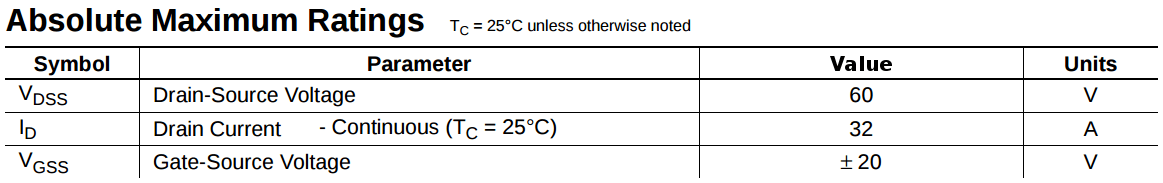• VDSS is the maximum voltage that is allowed between the `DRAIN` and the `SOURCE`.
Since our input only +12V even before the load (our lights) this is 5 times higher than we should ever expect to see.
Always ensure that your maximum `DRAIN`-`SOURCE` voltage is higher than any input voltage the device will ever see.
• ID is the maximum amount of current in amperes that can safely pass through the MOSFET at 25ºC.
This value will tend to go down as the temperature of the MOSFET goes up.
• VGSS is the maximum allowable `GATE`-`SOURCE` voltage the device can tolerate.
In this case anywhere between -20 volts and +20 volts.
Since our microcontroller can only reach about +3.3V we should be well within this range.

## Puzzling Particulars

Unless you happen to be a genius science cat this is likely all terribly confusing.
Don't beat yourself up about it.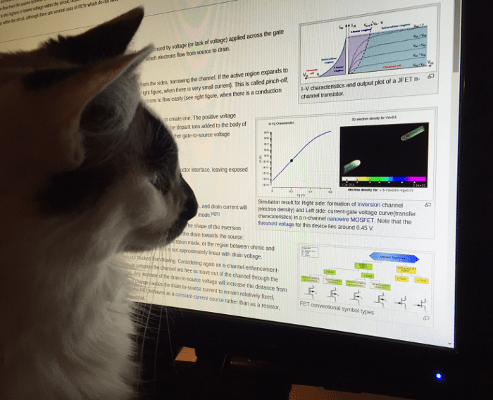The reality of what is going on here and why is far, far more complicated anyhow.

The important thing to know is that we will be looking at an N-channel Logic-level Enhancement-mode Power MOSFET.
Let's break down what this means from what we've learned so far:

• N-Channel: Positive charge will flow from the `DRAIN` pin to the `SOURCE` pin.
• Logic-level: The `GATE` is capable of being operated by the output of most microcontrollers.
• Enhancement-mode: The MOSFET is OFF by default.
• Power MOSFET: The MOSFET can handle enough power to switch a variety of loads.

That sounds simple enough now doesn't it?

## Concrete Characterization

Thus far everything discussed has been fairly abstract, so let's get real for a moment and discuss packages and pinouts.

MOSFETs come in a plethora of package shapes and sizes.
The package for the MOSFET on the GotT is known as a DPAK, TO-252, or TO-252-3.
While the basic functionality remains the same, there are some advantages to certain packages over others.

Here is a front and back view of a standard TO-220 package.If you are unsure of what package to choose, a TO-220 is generally a good choice for a number of reasons.

• It is a standard as defined by JEDEC. (The folks who standardize a lot of electronic components and functionality)
This means that it's not some proprietary package that you'll only be able to buy from one manufacturer.
• They have fairly large pins that are easy to solder by hand.
• The pitch of the pins is a convenient 2.54 mm which fits nicely in a breadboard or perfboard.
• It can dissipate more heat than smaller packages and therefore handle more power.
Typically up to a couple of watts without a heatsink.
• It has a nice mounting hole for a heatsink if things are getting too toasty.

One important note about TO-220 packages is that the Tab is often internally connected to the `DRAIN` for N-Channel MOSFETs and the `SOURCE` for P-Channel MOSFETs so take care not to short it to another pin unintentionally.

Remember that there is typically no downside to using a larger package than you require (other than the additional space it takes up) and it is cheap insurance to use a MOSFET that is larger than you need if you are uncertain.

One other thing to take note of when choosing a package is the pinout.

While many TO-220 packages have a `GATE-DRAIN-SOURCE` configuration to their pins, this is not always the case and many other packages will have different pinouts.
It is also not uncommon to find the same package with multiple different pinouts available.

Because of this, it is CRITICAL that you always, always check the pinout of your package in the datasheet.

Here are a few examples of the types of pinouts you might see.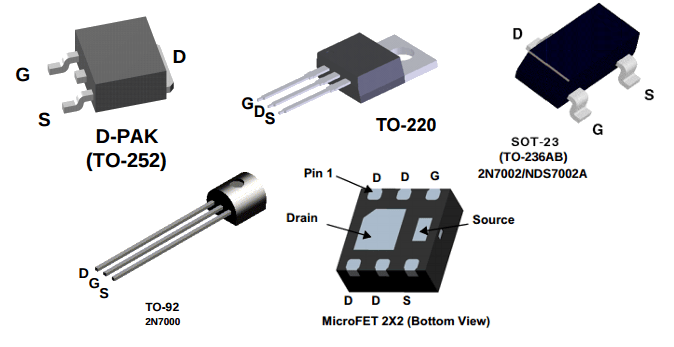### Schematic Symbols

If we're going to build a circuit we will need to create a schematic.
Here are a pair schematic symbols commonly used to represent N-Channel MOSFETs: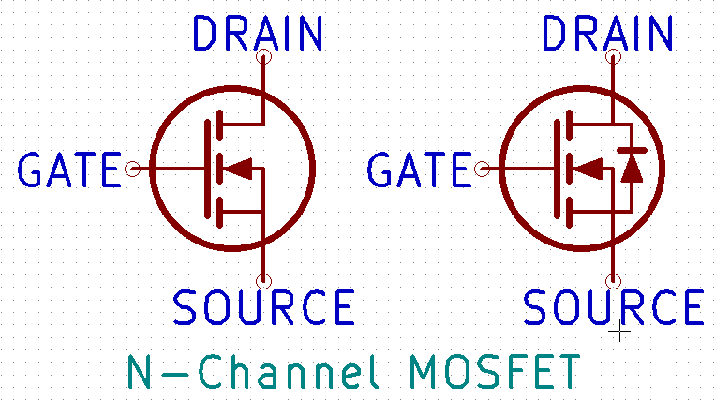Note that the `GATE`, `DRAIN`, and `SOURCE` labels have been added and will usually need to be inferred from the schematic symbol.

You may have noticed that the symbol on the right includes what appears to be a diode symbol between the `SOURCE` and `DRAIN`.
Sometimes this is shown as a regular diode, sometimes as a Zener diode, and sometimes not at all.

The fact of the matter is that this is not a separate device but rather a diode formed inherently by the way every MOSFET is made.
This is known as a Body Diode and while it often shows up on the schematic symbol for Power MOSFETs it is part of every MOSFET whether it is shown or not.
Because it's always there, there is no need to be particularly afraid of it. It's just handy to remember that it exists as it helps to explain some of the behavior of the MOSFET.

## Practical Prototype

This is all well and good but endless theory is moot without a practical example.So what's going on here?
The MOSFET we've covered but what are the two resistors doing there?

The 100Ω resistor between the microcontroller and the `GATE` (sometimes aptly called a Gate Resistor) serves primarily to reduce Ringing.
Ringing is an oscillation of voltage or current and is caused by the `GATE` capacitance in series with the connecting wire's inductance.
If you are switching something like lights this is probably not something you need be too terribly concerned about but it is cheap insurance.

This resistor can also help to protect the microcontroller from attempting to source too much current in some situations.
Most microcontrollers already limit the amount of current that any single pin can source but there is no reason to rely on this when a \$0.10 resistor can effectively negate both of the aforementioned issues.

The Internet is full of folks asking exactly what values these sort of resistors should be.
Sure, there is some ferociously complex math that will allow you determine the optimal range of values but outside of some very specific situations your time is almost certainly better spent elsewhere.

So does this resistor need to be exactly 100Ω?
Absolutely not. In fact, anywhere between 10Ω and a couple hundred Ω is probably just fine.
In a pinch you could probably even get away without it on a breadboard circuit but if you are designing a PCB it certainly won't hurt to have it there.

The 100KΩ resistor between the `GATE` and Ground is known as a Pull-Down Resistor.
It's job is simply to pull the `GATE` to Ground when the microcontroller isn't doing anything.
While many microcontrollers do have internal pull-up or pull-down resistors that can be set, what happens to your circuit when the microcontroller is turned off entirely?

When the state of a pin is not clearly defined it is known as Floating. When the `GATE` is left floating on our MOSFET there is really no way of knowing what it might be doing.
There's even the real possibility that the the MOSFET could switch ON and OFF at random when we do not want it to which could potentially be dangerous.
Except in some very specific applications, uncertainty is a bad thing in electronic circuits.
If the potential exists for an undefined behavior it is usually best to try to force it to a known state which is precisely what the pull-down resistor does; it pulls the `GATE` to Ground when nothing else is acting on it ensuring that the MOSFET remains OFF when it should be.

Does this resistor need to be exactly 100KΩ?
One again, the value is not critical and anything between about 1KΩ and 1MΩ will probably work just fine.
The larger the resistance, the slower the `GATE` will be pulled to Ground but unless we're trying to turn our circuit ON and OFF more than a thousand times a second (which for lights would just be silly) anything within the above range should work just fine.

While the exact value of either resistor is not critical, their values relative to one another can have some impact on the circuit.

### Algebraic Admonishment (MATH WARNING)

If you are somewhat familiar with electronics you may have noticed that the two resistors on the schematic form what is known as a voltage divider.

The formula for calculating the output of a voltage divider for the above circuit is:

VG = (VM x R2)/(R1 + R2)

Where:

• VG is the gate voltage (between the `GATE` pin and Ground) in volts (V): The term we are attempting to solve for
• VM is the source voltage (between the microcontroller pin and Ground) in volts (V): +3.3 (when the pin is HIGH)
• R1 is the resistance of R1 in Ohms (Ω): 100
• R2 is the resistance of R2 in Ohms (Ω): 100,000

Inserting the above terms into the equation yields:

VG = (3.3 x 100,000)/(100 + 100,000) ≈ +3.297V

3.297V is only .003V less than our original VM of +3.3V and still well above our maximum VGS(th) of +2.5V.

Regardless of what values you choose for R1 and R2 you will need to ensure that the voltage divider they form produces a voltage above VGS(th) sufficient for the MOSFET to turn ON.

### Switching Sides

So why did we build our circuit to look like the schematic on the left with our load between +12V and `DRAIN` instead of the one the right with our load between `SOURCE` and Ground?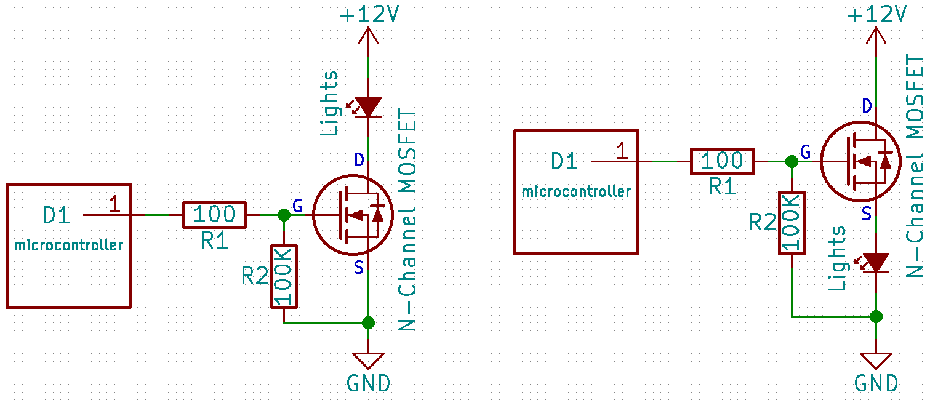Shouldn't they be the same? Does it make any difference?

The circuit on the left is known as a Low-side switching configuration, where the MOSFET acts as a current sink, and the circuit on the right is known as a High-side switching configuration, where the MOSFET acts as a current source.

As it turns out, there is a rather large difference between these two configurations and it all comes down to our friend the `GATE`-`SOURCE` threshold voltage or VGS(th).

We know that in order to turn ON our VGS must be at least VGS(th).
So let's calculate VGS for both of these circuits.

In the circuit on the left, we know from above that the `GATE` is at about +3.297V.
We also know that the `SOURCE` is at 0V because it is connected directly to Ground.
This calculation is very easy, resulting in a VGS of +3.297V.
Because +3.297V is above the maximum VGS(th) of +2.5V, the MOSFET will be turned ON when our microcontroller pin is HIGH.

The circuit on the right is slightly more difficult to calculate but nothing we can't handle.
In this case, the `GATE` is still at about +3.297V, so there's no problem there.
In order to calculate the voltage at the `SOURCE`, we need to account for the fact that the `SOURCE` is no longer connected directly to Ground (our lights are in the way).

For the sake of argument, let's assume that the `SOURCE` in this configuration was at 0V.
If that were the case, we would have the same situation as the low-side configuration and the MOSFET would turn on.
We know the MOSFET exhibits resistance when turned on and that this value is RDS(on).

With this in mind, the formula for calculating the voltage at the `SOURCE` is:

VS = (VCC x RL)/(RDS(on) + RL)

Where:

• VS is the voltage between the `SOURCE` pin and Ground in volts (V): The term we are attempting to solve for
• VCC is the source voltage (between the `DRAIN` pin and Ground) in volts (V): +12
• RL is the resistance of the load (our lights) in Ohms (Ω): Unknown
• RDS(on) is the resistance between the `DRAIN` pin and the `SOURCE` pin in Ohms (Ω): .045 (worst case)

We may not know the exact resistance of our load and for things like LEDs this can actually be a bit difficult to measure but for this calculation it's actually not a significant issue because of how small RDS(on) is by comparison.

Let's assume the resistance of our load was 10Ω:

VS = (12 x 10)/(.045 + 10) ≈ +11.95V

VS = (12 x 1000000)/(.045 + 1000000) ≈ +11.99V

With any reasonable load our voltage at the `SOURCE` pin is about +11.9V.
With that in mind, we can calculate VGS for this circuit to be:

VGS = +3.3V - +11.9V = -8.6V

...Wait a minute, VGS has to be greater than our VGS(th) of +2.5V in order to turn ON but it's -8.6V.
Simply put, the MOSFET will not be able to turn ON in this configuration unless we were able to get the `GATE` voltage to +14.4V!
Even with a VCC of +12V this would be pretty tough to accomplish.

Because of this the low-side configuration is almost always used for simply driving N-Channel MOSFETs.
A high-side configuration is commonly used with P-Channel MOSFETs and can be made to work with N-Channel MOSFETs using additional circuitry or `GATE` drivers though there is typically some specific design constraint requiring it if this is the case.

## Power Play

To understand how much power and thus heat our MOSFET will dissipate, let us first correct an all too common lack of attribution that proliferates even through modern texts with a very brief history lesson.

The German physicist and mathematician Georg Ohm first published Ohm's Law in his book Die galvanische Kette, mathematisch bearbeitet (tr., The Galvanic Circuit Investigated Mathematically) in 1827.
In circuit analysis, Ohm's Law is expressed as:

I = V/R or V = I x R or R = V/I

All of which are equivalent and where 'I' is the current in amperes, 'V' is the voltage in volts, and 'R' is the resistance in ohms.

James Prescott Joule, English physicist and brewer, was the first to study resistive heating and developed what would later come to be known as Joule's first law circa 1841.
Also called ohmic heating because of its relationship to Ohm's Law, Joule's formula for direct current heating is:

H ∝ I2 x R x t

Where 'H' is the heat generated in joules, 'I' is the current in amperes, 't' is the time in seconds, and '∝' is the symbol for direct proportionality.

In electronics we tend to use the watt (W) as a unit of power, named honorarily after Scottish engineer James Watt in 1882 for his development of the concept of horsepower with regard to his work on steam engines after his death in 1819.
The term was adopted in 1960 by the General Conference on Weights and Measures as an official SI unit. One watt is defined as:

W = 1J/s or 1 joule per second

Substituting this term into Joule's Law yields:

P = I2 x R

Where 'P' is the power in watts, 'R' is the resistance in ohms, and 'I' is the current in amperes. This is the commonly used expression for power in electronic circuits often misattributed with being entirely a part of Ohm's Law.

In reality, a number of factors go into determining the actual power dissipated by the MOSFET.
RDS(on) varies depending upon the current flowing from `DRAIN` to `SOURCE`, which can in turn be dependent upon the ambient temperature (RDS(on) typically increases with temperature), `DRAIN`-`SOURCE` voltage, `GATE` voltage, duty-cycle, conduction and switching losses, and a slew of other complex factors that can make this infinitely more complex to calculate.

We can, however, estimate approximate power using the above equation.
Since we know that 'R' (Using RDS(on) as an average) is about 35 milliohms or .035Ω we can solve the above equation for various amounts of current we might sink.
Let's assume our LEDs or load draw 1A, 5A, 10A, and the absolute maximum our MOSFET is rated for 32A.

P = 12 x .035 = .035W (No problem)

P = 52 x .035 = .875W (Probably fine without a heatsink)

P = 102 x .035 = 3.5W (A heatsink is a good idea or things are likely to get a little toasty)

P = 322 x .035 = 35.84W (Large heatsink or fire, your choice)

## Design Dossier

I hope that you have enjoyed, or at least learned a little, during this brief foray into the world of MOSFETs.

All of the design files and BOM for this board can be found on the GotT-Translation-Circuit GitHub page and boards may be ordered here: